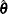Iterative Algorithms for Model Fitting

Two iterative maximum likelihood algorithms are available in PROC SURVEYLOGISTIC to obtain the pseudo-estimateof the model parameter. The default is the Fisher scoring method, which is equivalent to fitting by iteratively reweighted least squares. The alternative algorithm is the Newton-Raphson method. Both algorithms give the same parameter estimates; the covariance matrix ofis estimated in the section Variance Estimation. For a generalized logit model, only the Newton-Raphson technique is available. You can use the TECHNIQUE= option in the MODEL statement to select a fitting algorithm.